Home Practice
For learners and parents For teachers and schools
Textbooks
Full catalogue
Pricing SupportLog in

We think you are located in United States. Is this correct?

# End of chapter exercises

## End of chapter exercises

Textbook Exercise 5.33

Show that if $$a<0$$, then the range of $$f(x)=a{\left(x+p\right)}^{2}+q$$ is $$\left\{f(x):f(x)\in \left(-\infty ,q\right]\right\}$$.

\begin{align*} (x + p)^2 \geq 0\\ a(x + p)^2 \leq 0 \\ a(x + p)^2 + q < q \\ \therefore f(x) < q \end{align*}

If $$\left(2;7\right)$$ is the turning point of $$f(x)=-2{x}^{2}-4ax+k$$, find the values of the constants $$a$$ and $$k$$.

$$a = -2; k= -1$$

The following graph is represented by the equation $$f(x)=a{x}^{2}+bx$$. The coordinates of the turning point are $$\left(3;9\right)$$. Show that $$a=-1$$ and $$b=6$$.\begin{align*} f(x) & = ax^2 + bx \\ x &= -{b}{2a} \\ \therefore 3 &= -\frac{b}{2a}\\ 6a &= -b\\ \text{subst. } (3;9) \quad 9 &= a(3)^2 + b(3) \\ 9 &= 9a + 3b \\ 9 &= 9a + 3(-6a) \\ 9 &= 9a -18a \\ 9 &= -9a \\ \therefore -1 &= a \\ \therefore b &= 6 \end{align*}

Given: $$f(x)={x}^{2}-2x+3$$. Give the equation of the new graph originating if:

the graph of $$f$$ is moved three units to the left.

$$y={x + 2}^{2} + 2$$

the $$x$$-axis is moved down three units.

$$y={x - 1}^{2} + 5$$

A parabola with turning point $$\left(-1;-4\right)$$ is shifted vertically by $$\text{4}$$ units upwards. What are the coordinates of the turning point of the shifted parabola?

$$(-1;0)$$

Plot the graph of the hyperbola defined by $$y=\frac{2}{x}$$ for $$-4\le x\le 4$$. Suppose the hyperbola is shifted $$\text{3}$$ units to the right and $$\text{1}$$ unit down. What is the new equation then?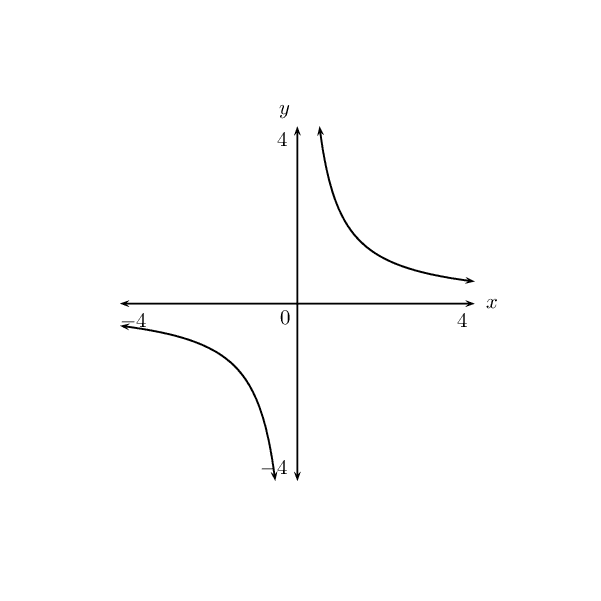Based on the graph of $$y=\frac{k}{(x+p)} + q$$, determine the equation of the graph with asymptotes $$y=2$$ and $$x=1$$ and passing through the point $$\left(2;3\right)$$.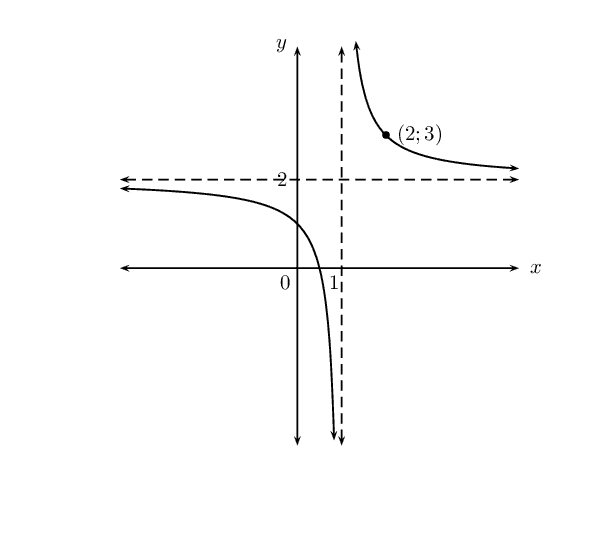$$y=\frac{1}{(x-1)} + 2$$

The columns in the table below give the $$y$$-values for the following functions: $$y={a}^{x}$$, $$y={a}^{x+1}$$ and $$y={a}^{x}+1$$. Match each function to the correct column.

 $$x$$ A B C $$-\text{2}$$ $$\text{7,25}$$ $$\text{6,25}$$ $$\text{2,5}$$ $$-\text{1}$$ $$\text{3,5}$$ $$\text{2,5}$$ $$\text{1}$$ $$\text{0}$$ $$\text{2}$$ $$\text{1}$$ $$\text{0,4}$$ $$\text{1}$$ $$\text{1,4}$$ $$\text{0,4}$$ $$\text{0,16}$$ $$\text{2}$$ $$\text{1,16}$$ $$\text{0,16}$$ $$\text{0,064}$$

Column A: $$y={a}^{x} + 1$$; Column B: $$y={a}^{x}$$; Column C: $$y={a}^{x + 1}$$

The graph of $$f(x) = 1 + a \cdot 2^x$$ ($$a$$ is a constant) passes through the origin.

Determine the value of $$a$$.

\begin{align*} 0 &= a \times 2^0 + 1 \\ \therefore -1 &= a \end{align*}

Determine the value of $$f(-15)$$ correct to five decimal places.

\begin{align*} f(-15) &= -2^{-15} + 1 \\ &= \text{0,99997} \end{align*}

Determine the value of $$x$$, if $$P\left(x;\text{0,5}\right)$$ lies on the graph of $$f$$.

\begin{align*} \text{0,5} &= -2^{x} + 1 \\ \text{0,5} &= 2^{x} \\ \therefore x &= -1 \end{align*}

If the graph of $$f$$ is shifted $$\text{2}$$ units to the right to give the function $$h$$, write down the equation of $$h$$.

$$h(x) = -2^{(x -2)} + 1$$

The graph of $$f(x) = a \cdot b^x$$ ($$a ≠ 0$$) has the point $$P\left(2;\text{144}\right)$$ on $$f$$.

If $$b = \text{0,75}$$, calculate the value of $$a$$.

$$a = 256$$

Hence write down the equation of $$f$$.

$$f(x) = 256 \left( \frac{3}{4} \right)^x$$

Determine, correct to two decimal places, the value of $$f(13)$$.

$$f(13) = \text{6,08}$$

Describe the transformation of the curve of $$f$$ to $$h$$ if $$h(x)=f(-x)$$.

Shifted $$\text{2}$$ units to the right

Using your knowledge of the effects of $$p$$ and $$k$$ draw a rough sketch of the following graphs without a table of values.

$$y = \sin 3\theta$$ for $$-\text{180}\text{°} \leq \theta \leq \text{180}\text{°}$$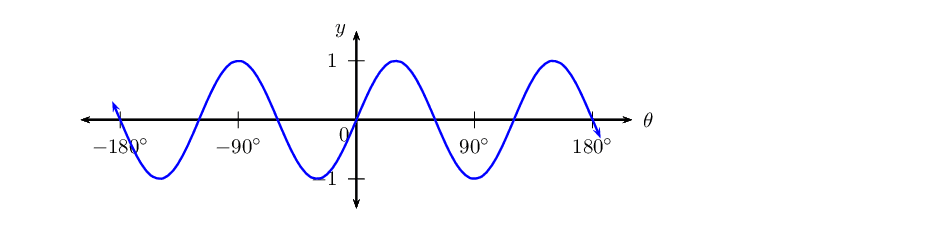$$y = -\cos 2\theta$$ for $$\text{0}\text{°} \leq \theta \leq \text{180}\text{°}$$$$y = \tan \frac{1}{2}\theta$$ for $$\text{0}\text{°} \leq \theta \leq \text{360}\text{°}$$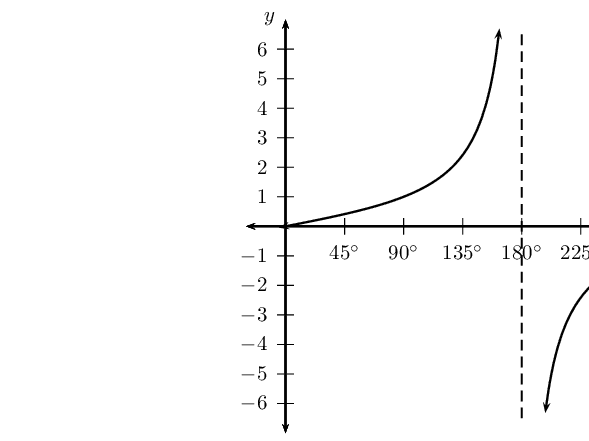$$y = \sin (\theta-\text{45}\text{°})$$ for $$-\text{360}\text{°} \leq \theta \leq \text{360}\text{°}$$$$y = \cos (\theta+\text{45}\text{°})$$ for $$\text{0}\text{°} \leq \theta \leq \text{360}\text{°}$$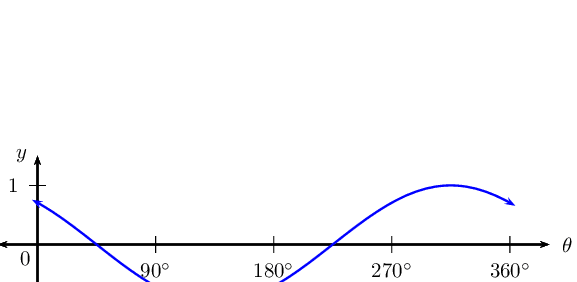$$y = \tan (\theta-\text{45}\text{°})$$ for $$\text{0}\text{°} \leq \theta \leq \text{360}\text{°}$$$$y = 2\sin 2\theta$$ for $$-\text{180}\text{°} \leq \theta \leq \text{180}\text{°}$$$$y = \sin (\theta+\text{30}\text{°}) + 1$$ for $$-\text{360}\text{°} \leq \theta \leq \text{0}\text{°}$$